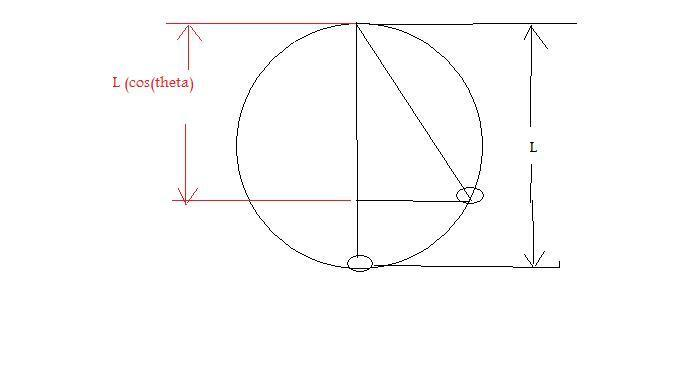# Find the speed at the bottom of it's swing

• Dark Visitor

#### Dark Visitor

A simple pendulum, 2 m in length, is released with a push (i.e. - it has an initial velocity) when the support string is at an angle of 25 degrees from the vertical. If the initial speed of the suspended mass is 1.2 m/s when at the release point, use conservation of energy to find it's speed at the bottom of the swing.

* 2.3 m/s
* 2.6 m/s
* 2 m/s
* .5 m/s

I don't really understand how to use conservation of energy that well, so I need help. How would I apply that for this problem? And how do I solve from there? Any help would be much appreciated. I need it by tomorrow night. Thanks.

Do you understand what would happen if there were no push? Energy is continually transferred back and forth between kinetic and potential, but the sum is constant at all times.

First, find the height of the bob above its position at the bottom from the geometr, ie the vertical displacement between 25 degrees and vertical.

Keep track of what the item's total energy is (PE+KE) and this cannot change. At the bottom of the swing all the energy is kinetic (since it's convenient to define PE=0 at the bottom of the swing in this case). :)

What do you mean by the bob and geometer? And I would think the height would always remain 2 m due to the length.

To Matterwave: You're saying that at the bottom when PE = 0, that KE will still equal all of the energy that was there when PE did not equal 0?

What do you mean by the bob and geometer? And I would think the height would always remain 2 m due to the length.

To Matterwave: You're saying that at the bottom when PE = 0, that KE will still equal all of the energy that was there when PE did not equal 0?

er sorry, that was meant to be geometry and the bob is just the mass on the end. The change in height is given by L(1-cos(25)). Can you see why?

The kinetic energy at the bottom will be the sum of the kinetic energy from the push plus the potential energy from it being displaced by 25 degrees, see above.

It's okay. Well, I see that the cos25 gives me the length on the "x axis" of the pendulum when it is at the 25 degree angle, but I don't know where the 1 comes in, or what L stands for, unless it is length.

It's okay. Well, I see that the cos25 gives me the length on the "x axis" of the pendulum when it is at the 25 degree angle, but I don't know where the 1 comes in, or what L stands for, unless it is length.
Right L is length = 2m. I'll post a pic here in a minute.

Okay, I think I understand a little more. So the length won't be 2 meters anymore. It will be:
2 m(1 - cos25), which makes it a lot smaller.

My question now is how does this help me get the final speed?The displacement of the bob vertically is given by L - L(cos(25))=L(1-cos(25))

The potential energy is mgh where h is described as L(1-cos(25)). The total energy is the initial kinetic from the push (1/2mV^2) + the potential energy (mgh). That do it?

Yes, quite a bit. One thing I still don't get is how we can use either of those when we don't have the mass (m) for the equations. Do we assume anything, or do we need to find it?

OOps, I forgot what we we're trying to calculate. Well since all masses fall at the same rate, it doesn't matter. Just add the velocity it would have attained if dropped from that height. Different ways to get this, but V'^2=V^2+2(g*h) is the most straight froward at this point. V' is the final velocity, v is the initial.

Okay:

vf2 = vi2 + 2(g)(h)
= (1.2)2 + 2(9.8)(.1874)
= 5.11304

Then I square-rooted that and got 2.2612, which 2.3 m/s is an answer.

Good job.

Thanks, and thanks for all the help. I really appreciate it. If you have some free time to spare, please help me on some of my other posts. I got a lot of them, and not many people helping me. If you can't, it's fine. Thanks.

Thanks, and thanks for all the help. I really appreciate it. If you have some free time to spare, please help me on some of my other posts. I got a lot of them, and not many people helping me. If you can't, it's fine. Thanks.

Sure I'll look for them--must be a sunday, things are humming here. ;-D

Yeah. I posted most yesterday to allow time for people to see them and respond, but didn't happen too much. Fortunately, I still have time to complete them all.